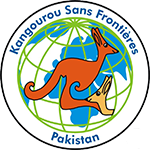## Kangaroo-Junior Test Preparation

### Unlimited Printable Worksheets, Online Practice and Online Tests For Kangaroo-Junior• The duration of the competition is 3 hours.
• 30 problems to solve: ten – 3 points each, ten – 4 points each and ten – 5 points each.
• Calculators are not allowed. You may use basic geometric tools.
• Scope of problems:
• operations on real numbers.
• functions, polynomials, equations, inequalities.
• sequences of numbers.
• elements of combinatorics.
• synthetic plane geometry.
• analytic plane geometry.
(Source : http://ikmc.kangaroo.org.pk/ )
Coordinate Geometry, Algebra, Mensuration - Perimeter, Area, Volume, etc., Data Handling, Number System, Geometry, Heron's Formula, Quadrilaterals, Logical Reasoning, Linear Equations in Two Variables, . . .

Trigonometry, Arithmetic Progressions, Data Handling, Real Numbers, Logical Reasoning, Quadratic Equations, Linear Equations in Two Variables, Algebra, Mensuration - Perimeter, Area, Volume, etc., Geometry, . . .